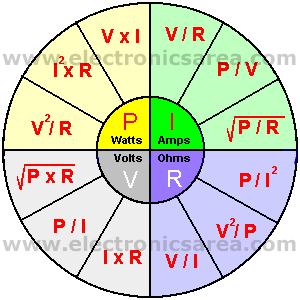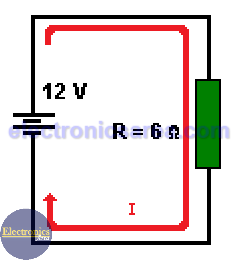# Ohm’s Law and the Electric Power

Ohm’s Law relates in one equation the voltage, the current and resistance. Resistance = resistor’s value.

A more complete expression of Ohm’s Law is achieved using the electric power formula. Using the known formula of power: P = VxI, (Power = voltage x current), and its variants: V = P/I and I = P/V, additional formulas are obtained.

These new equations allow us to obtain the values of power, voltage, current and resistance, with only two of the four variables.

• Solving for P, we get: P = V2/R, P = I2 x R, P = V x I (watts)
• Solving for I, we get: I = V/R, I = P/V, I = (P/R)1/2 (amps)
• Solving for R, we get: R = V/I, R = V2/P, R = P/I2 (ohms)
• Solving for V, we get: V = (PxR)1/2, V = P/I, V = IxR (volts)

The following diagram shows a complete summary of the formulas, arranged so that it is easier to memorize.Note: The square root = ( )1/2.

Problem: Find the power disipated on the resistor R = 6 ohms connected to a battery of 12 volts.1- Solving the problem using the formula: P = I2xR

The electric current flowing through the circuit is: I = 12 volt/6 ohms = 2 amperes. We can obtain the power disipated on heat on the resistor using the formula: P = I2xR = 22x6 = 24 watts

2 – Solving the problem using the formula: P = V x I

We can find the power on the resistor using the formula: P = VxI = 12 x 2 = 24 watts

3 – Solving the problem using the formula: P= V2/R

In the same way, we can find the power on the resistor using the formula: P = V2/R = 122 / 6 = 24 watts. Using this formula, you don’t need to find the current flowing through the circuit.

• 3
•
•
•
•
•
•
•
3
Shares
•
3
Shares
• 3
•
•
•
•
•
•